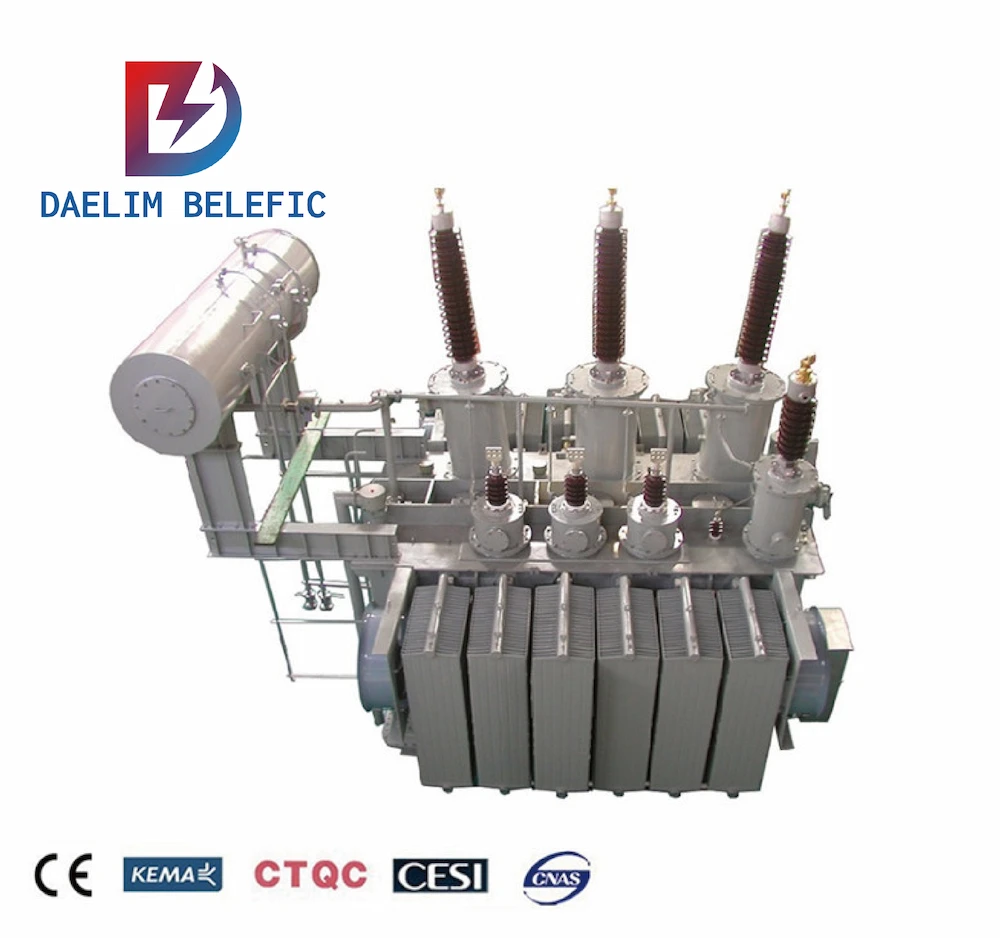# Selection Method of Distribution Power Transformer

We can provide you single phase and three phase pad mounted transformer

#### Dry-type Transformer

Type：Cast resin; Rated Capacity: Up to 25MVA; Rated Voltage: Up to 36KV;

#### Pole Transformer

TypeCSP type Frequency: 50/60Hz; Rated Power: 5~167kva

#### Dry-type Transformer

Frequency: 50/60Hz Rated voltage:10kv, 20kv,30kv Rated Power: 400~2500kva

## 1. Equation

Selection Distribution Power Transformer capacity = total electrical equipment X simultaneous rate/power factor + efficiency.

How To Choose The 10kv Transformer Capacity Grade

## 2.Description

The total electrical equipment refers to the sum of the power of all equipment in the factory, and the simultaneous rate refers to the ratio of the actual capacity of the equipment put into operation at the same time to the capacity of the electrical equipment. It is generally chosen to be about 0.7.

##### Get the lastest daelim transformer product cataloge now## 4. Efficiency: 0.85-0.9.

Distribution Power Transformer capacity = total capacity of electrical equipment × simultaneous rate / power factor of electrical equipment × efficiency of electrical equipment

Example: In a factory, the total capacity of electrical equipment is 700KVA, and the actual consumption is 600KVA. Find the capacity of the power transformer.

At the same time, the power is 600KVA÷700KVA=0.86, and the power transformer capacity=(700×0.86)/(cosΦ=0.85×0.85)=600/0.722=830KVA.

Note: If the power factor is 0.95 and the efficiency is 0.9, its capacity is: Distribution Power Transformer capacity=(700×0.7)/(cosΦ=0.95×n=0.9)=490/0.855=576KVA, such as: power factor=0.7, n =0.9 The simultaneous rate is 0.75, find the capacity.

Power transformer capacity=(700KVA×0.75)/(0.7×0.9)=525/0.63=833KVA.

It can be seen that the lower the power factor, the greater the selection of Distribution Power Transformers, and vice versa, the power factor must be improved to achieve the purpose of energy saving and consumption reduction.

### 10 kv Oil Filled Transformer

Fault Diagnosis and Analysis of 10 kv Oil Filled Transformer 10 kv Oil filled transformer is a key equipment for power distribution, and its failure